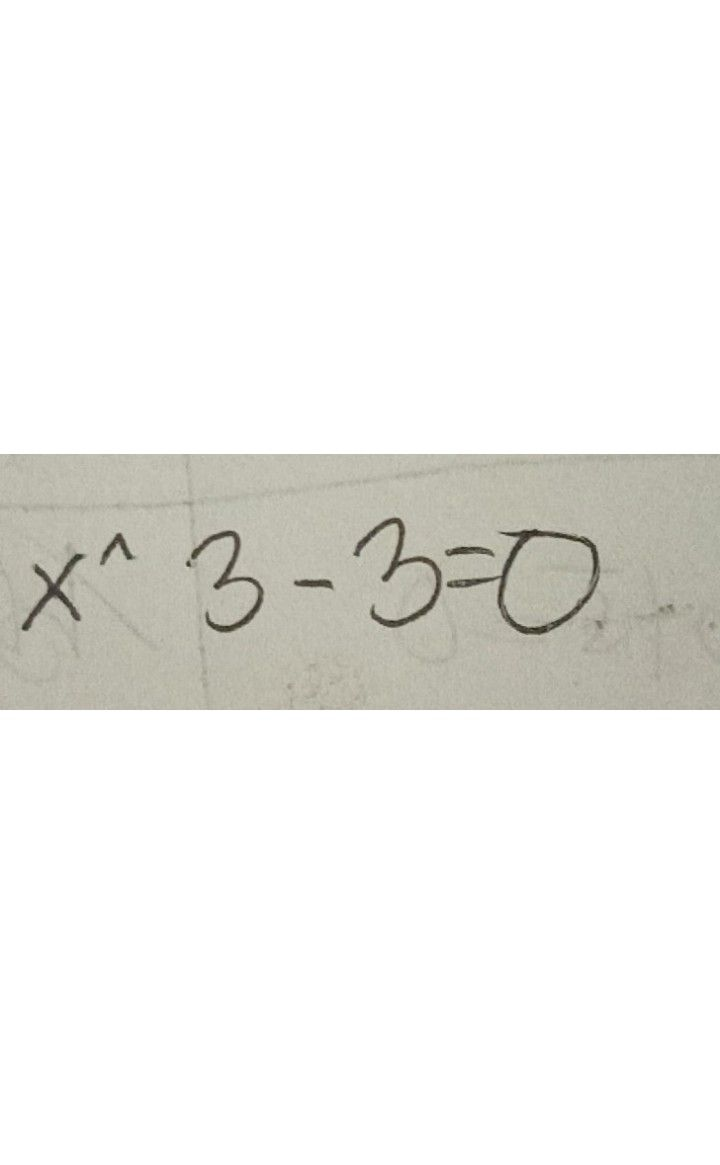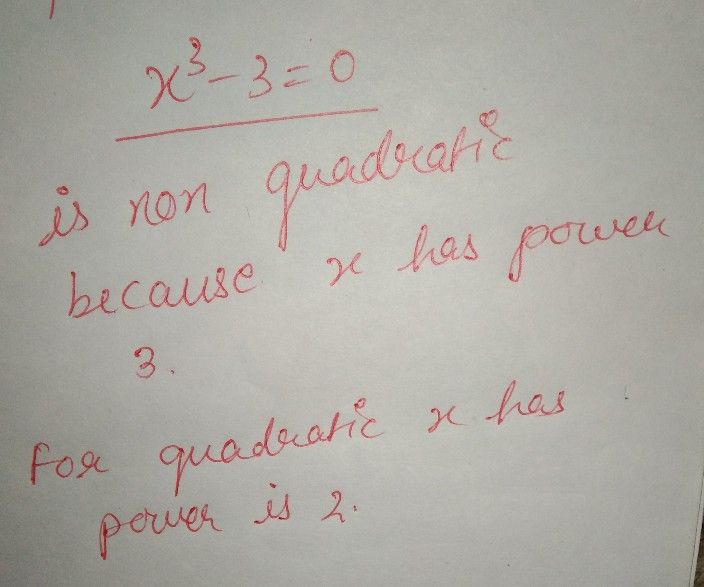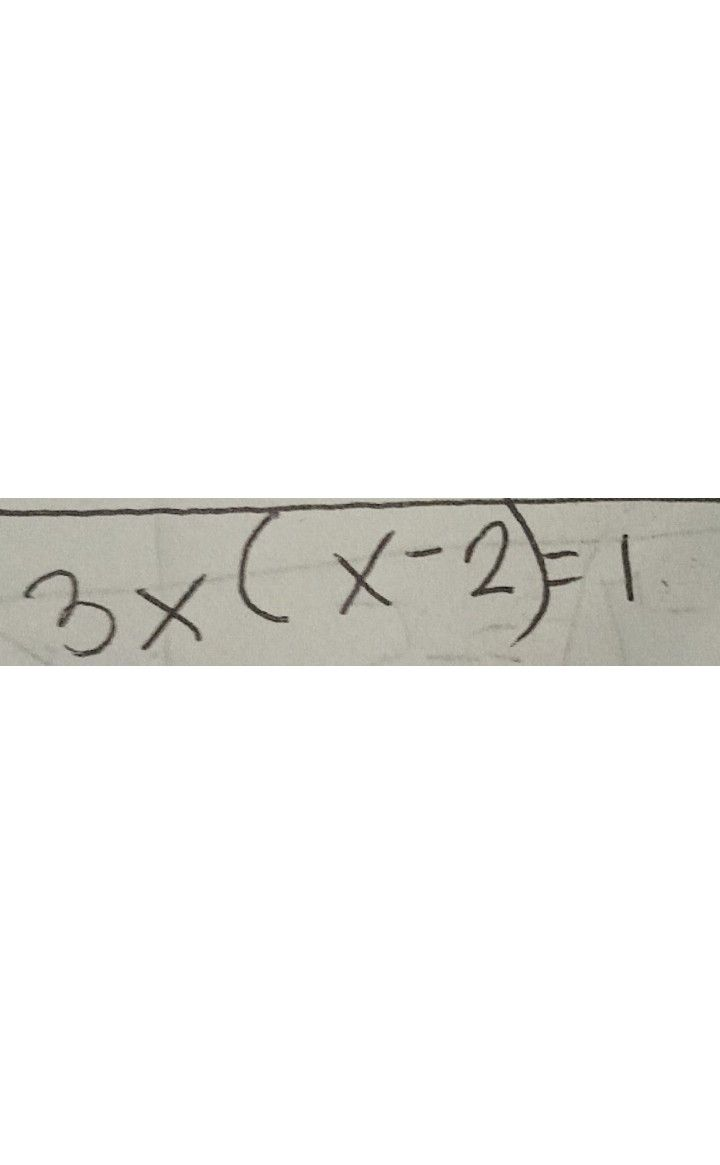Symbol
Problem$5\times n_{2+2x+3}=0$
Algebra
Question content
SolutionQanda teacher - sanas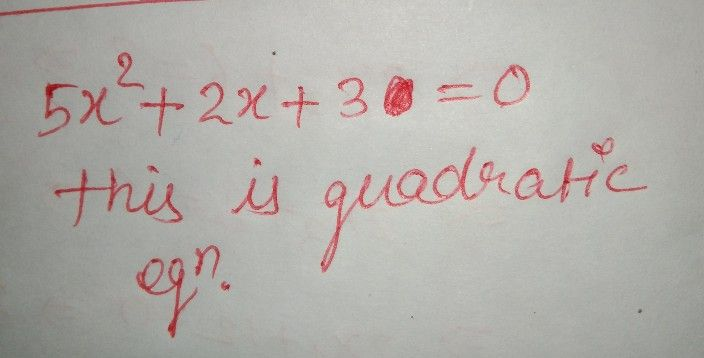any doubt pls ask.. if u like my ans pls give me 5 star rating.......Student
ahmm sorry i ask if the equation is quadratic or non-Quadratic. you just need to choice to the twoQanda teacher - sanas
what happenStudent
you just need to choice its either quadratic or non Quadratic and then the equation is at the pic that i sentQanda teacher - sanas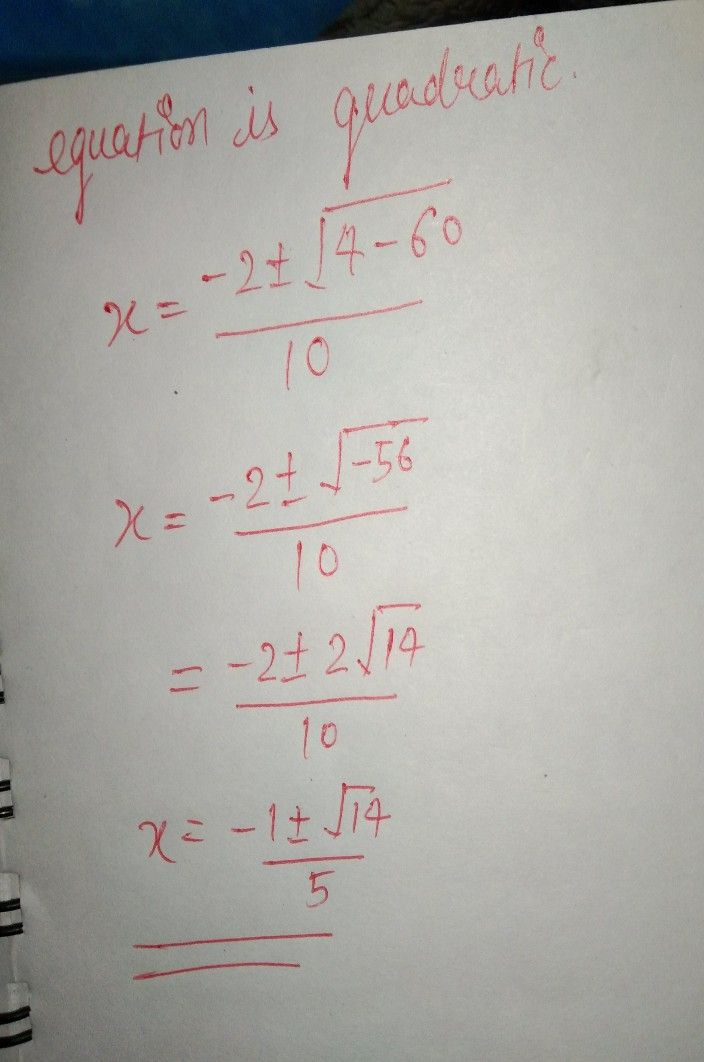Student
do you mean, the pic that i sent to you is an quadratic? or non-Quadratic?Qanda teacher - sanasStudent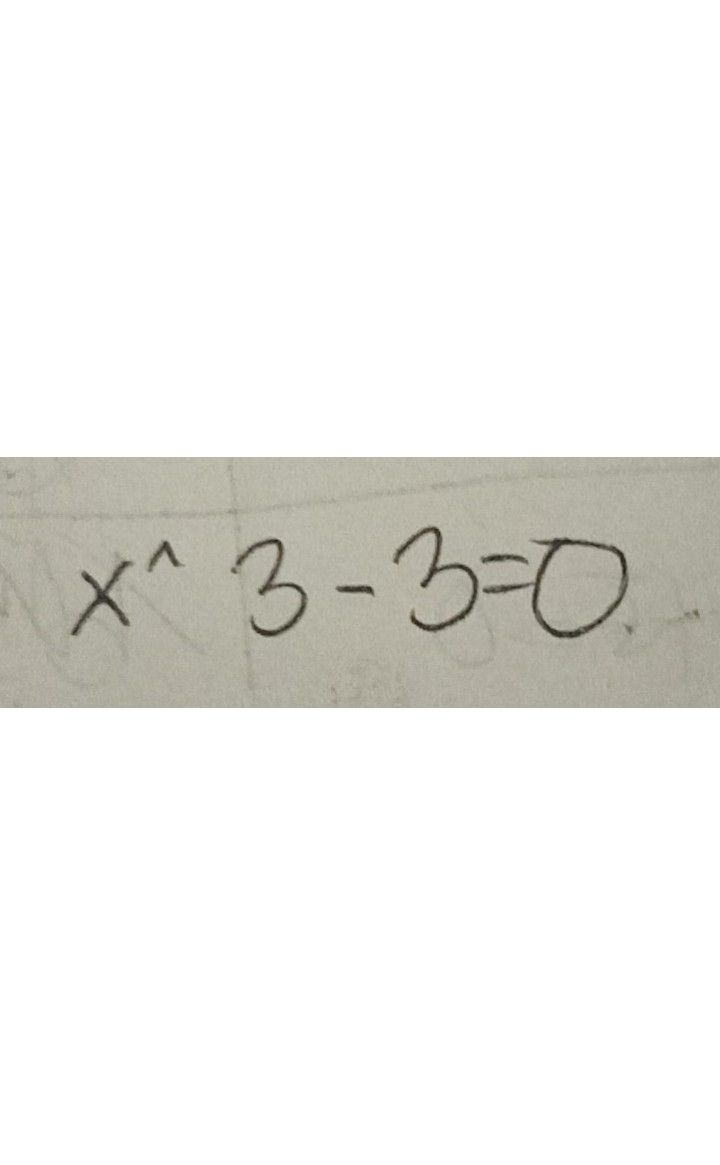Qanda teacher - sanas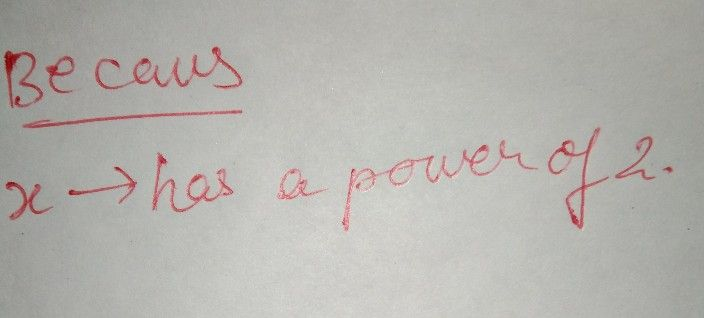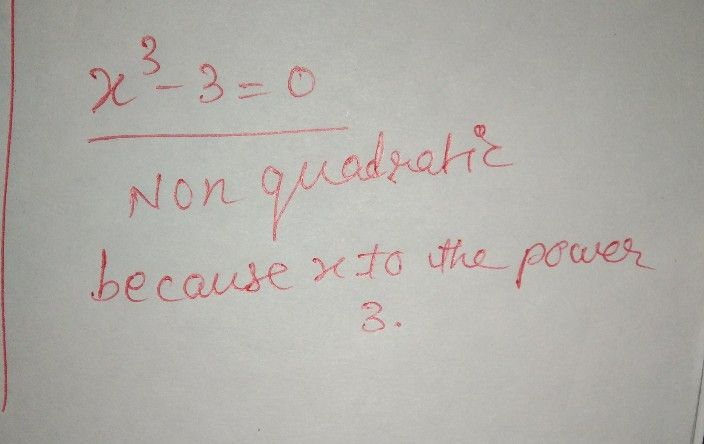Student